Mathematics
Easy

Question

# The linear equation that have slope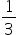is

## y = 3xy = -3x3y = x3y = -xHint:

## The correct answer is: 3y = x

### In the question the slope of a equation of line isWe have to find the equation of the line having slop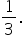In option a the equation isy=3x       .............(1)On comparing eq 1 with y=mx+c we get, m=3 and c=0.Here, the slope of the line is 3.In option b the equation of line isy=-3x            ................(2)On comparing eq 2 with y=mx+c we get, m=-3.Here, the slope of the line is -3.In option c the equation of line is3y=x              ................(3)Convert eq 3 in the form of y=mx+c.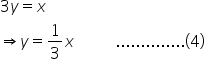On comparing eq 4 with y=mx+c we get, m=Here, the slope of the line isIn option d the equation of line is3y=-x           ..............(5)Convert eq 5 into the form y=mx+c.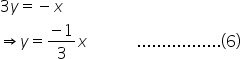On comparing eq 6 with y=mx+c, we get m=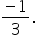Here, the slope of the line is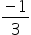.So, the equation having slopeis 3y=x.Therefore the correct option is c, i.e., 3y=x.

The standard equation of line is y=mx+c, where m is the slope of the line and c is the intercept. To write the equation of a line we need to find the value of slope and the intercept.#### With Turito Foundation.#### Get an Expert Advice From Turito.